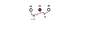# Center of mass of a spring

• aaaa202

#### aaaa202

A week ago I posted a thread about my conceptual understanding of the center of mass of a body. I have however not yet gained the intuition that I want, so let me ask a question about the center of mass of a spring.

Consider a spring which is elongated in outer space and left to oscillate. As we know from intuition the spring will oscillate such that the middle point on it stands still. My question is:
Is this because the middle point is the center of mass?

At first I thought well of course but then I became doubtful. This is because from my understanding, the center of mass is not really a physical point on the spring - that is you can't take a spring and then point on the atom in the middle of it and say that it's the center of mass. The center of mass is a weighted average of all the position vectors of each atom in the spring. Thus it is a more abstract point which is associated with the coordinate frame in which you represent the spring, not the physical spring itself. Nonetheless you can actually point to a physical part of the spring and say: This stands still. So why is that?

I can try explain in another way: I understand the center of mass and its applications when dealing with point charges in vacuum. Then the center of mass is just an abstract mathematical point. But when you are dealing with a continuous body it suddenly seems to become a physical point on that body.

As we know from intuition the spring will oscillate such that the middle point on it stands still. My question is:
Is this because the middle point is the center of mass?

"Intuition is what tells us the Earth is flat." --anon

I would agree that if you had a spring in space, oscillating along the x-axis (centered, say, at the origin), that there will be one point on the spring that would appear to not be moving, as long as you are viewing it in the y-z plane (at x = 0).

What might you expect to see of you were on the x-axis (inside the spring) looking towards the origin?

Last edited:
I don't really understand what you mean but I think I found the solution myself. See you can't really say in general that because the center of mass lies in the middle of a physical body that the physical particles at that point will stand still. Consider for instance the drawing attached. Clearly the center of mass is in the middle, i.e. where the red particle is. The motion of this particle will only coincide with the motion of the center of mass IF the force on the red particle due to one of the two other particles is the same as the other. This doesn't necessarily have to hold. Imagine that the particles had identical mass but different amounts of charge on them, e.g. -1,-1,-2. Then the center of mass would not move but the particle in the middle would.

#### Attachments

•centerofmas.png
2.1 KB · Views: 415
I don't really understand what you mean but ...
I'll be more specific--the center of mass in this case is in the middle of the spring, just not on the spring.

Then the center of mass would not move but the particle in the middle would.
I would think that if the mass distribution of your 3-particle structure changes, so would the center of mass.

I would think that if the mass distribution of your 3-particle structure changes, so would the center of mass.

What? There's no external forces in the system? When planets move relative to each other the mass distribution changes, but that doesn't mean their center of mass should change - though it could be moving with uniform velocity.

My point was really just that the reason why the middle point ON the spring does not move actually has little to do with it being the center of mass.